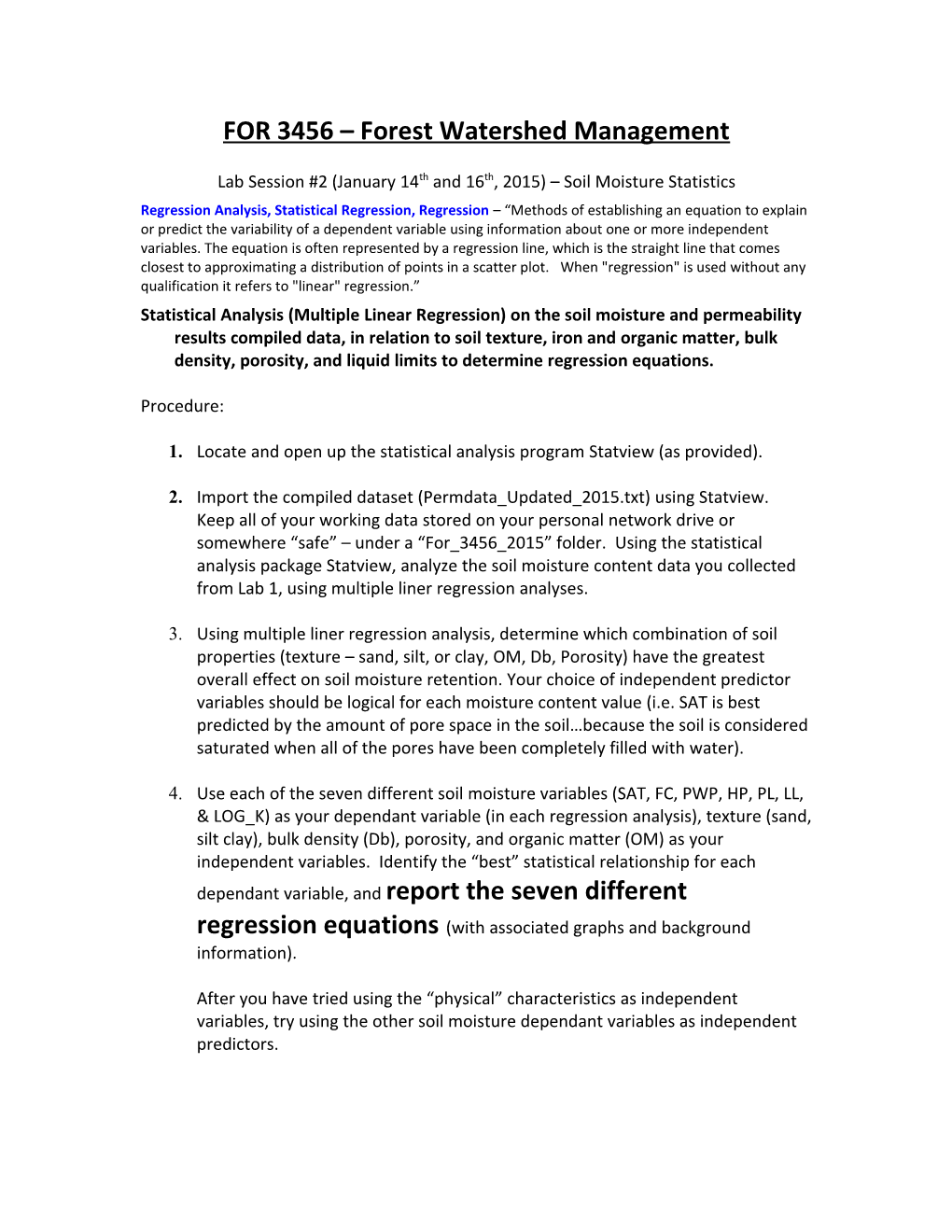# FOR 3456 Forest Watershed & Forest Fire ManagementFOR 3456 – Forest Watershed Management

Lab Session #2 (January 14th and 16th, 2015) – Soil Moisture Statistics

Regression Analysis, Statistical Regression, Regression – “Methods of establishing an equation to explain or predict the variability of a dependent variable using information about one or more independent variables. The equation is often represented by a regression line, which is the straight line that comes closest to approximating a distribution of points in a scatter plot. When "regression" is used without any qualification it refers to "linear" regression.”

## 2.Import the compiled dataset (Permdata_Updated_2015.txt) using Statview. Keep all of your working data stored on your personal network drive or somewhere “safe” – under a “For_3456_2015” folder. Using the statistical analysis package Statview, analyze the soil moisture content data you collected from Lab 1, using multiple liner regression analyses.

1. Using multiple liner regression analysis, determine which combination of soil properties (texture – sand, silt, or clay, OM, Db, Porosity) have the greatest overall effect on soil moisture retention. Your choice of independent predictor variables should be logical for each moisture content value (i.e. SAT is best predicted by the amount of pore space in the soil…because the soil is considered saturated when all of the pores have been completely filled with water).
1. Use each of the seven different soil moisture variables (SAT, FC, PWP, HP, PL, LL, & LOG_K) as your dependant variable (in each regression analysis), texture (sand, silt clay), bulk density (Db), porosity, and organic matter (OM) as your independent variables. Identify the “best” statistical relationship for each dependant variable, and report the seven different regression equations(with associated graphs and background information).

After you have tried using the “physical” characteristics as independent variables, try using the other soil moisture dependant variables as independent predictors.

1. Once you have derived the “best” regression relationships for each of the seven dependant variables, save a new column of data in your data spreadsheet for each (predicted values) based on the following step.
1. For each of your final chosen regression analyses, save the “fitted values”…so that you can plot the actual data vs. the predicted values. When you are working in the analysis window, the fitted / predicted values will appear as a new column of data on your working spreadsheet (far right column).
1. Determine which combination of these soil physical properties had the greatest influence on soil moisture retention using all the samples analyzed.
1. Use scatter plots of your regression data to view trends.

Refer to the following page for an example of the scatterplot and regression equation design.

Deadline for the first report will be one week from today (Wednesday / Friday, January 21st / 23rd, 2015 – 5:30pm).

For 3456 – Lab Session #2

Statistical Regression Work – Multiple Linear Regression Analysis

Dataset being used – Permdata_Updated_2015.xlsx / Permdata_Updated_2015.txt

Purpose:

To analyze the compiled dataset using multiple linear regression, and derive the “best” (relating to the minimum # of independent variables used, highest r² value gained…etc.) regression equations for all seven (7) dependant variables (SAT, FC, PWP, HP, PL, LL, Log K).

Produce regression scatterplots of the analyzed data for each by saving the “unstandardized predicted values” (i.e. predicted dependant values generated from regression analysis), and then graphing the “Actual” (Y axis) versus “Predicted” (X Axis) data.

EXAMPLE